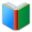# List 1 for 7th Grade MATH

Middle School Words: List 1 for 7th Grade MATH, random words are selected for the Word Quiz worksheet. This sheet is created dynamically, every sheet is different from previous ones. You can download and print the worksheet from browser directly.
 Actions upon current list

 All lists of current grdae
Spelling Words:

Arts Words:

Social Words:

Math Words:

Science Words:

Literature Words:The Shoes of FortuneThe ShadowThe Adventures of Sherlock HolmesAdventures of Huckleberry Finn
 Worksheet - Middle School Words: List 1 for 7th Grade MATH

 Print: List 1 for 7th Grade MATH

Exam Word - https://www.examword.com/

Middle School Words: List 1 for 7th Grade MATH

 1: the angle between adjacent sides of a rectilinear figure isosceles triangle[__] interior angles[__] right triangle[__] negative[__] 2: a polygon with all sides and all angles equal exterior angles[__] regular polygon[__] right triangle[__] interest[__] 3: when two lines are crossed by another line, the angles in matching corners interest[__] regular polygon[__] unit rate[__] corresponding angles[__] 4: rearrangement of the elements of a set isosceles triangle[__] corresponding angles[__] permutations[__] regular polygon[__] 5: a triangle that contains an obtuse interior angle obtuse triangle[__] vertical angle[__] scale factor[__] discount[__]